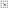## Journal directory listing - Volume 21-30 (1976-1985) - Volume 30 (1985)Directory Top

##### On the Chromatic Polynomials of Hypergraphs
Author: Nai-Hung Hsu

Abstract：

In this paper, we define and develop the properties of the strongly chromatic polynomials and weakly chromatic polynomials of hypergraphs. We prove that strongly chromatic polynomials satisfy the fundamental theorem. We also prove that if H1 and H2 are two q-edge-tree r-uniform hypergraphs, then H1 and H2 are both strongly chromatically equivalent and weakly chromatically equivalent, and we show that if H1 and H2 are connected nested hypergraphs with the same order, then H1 and H2 are strongly chromatically equivalent.